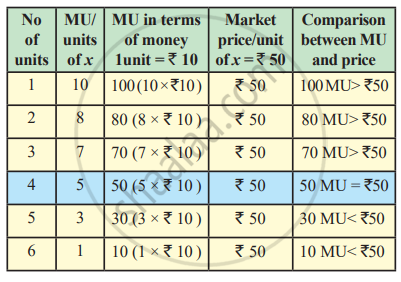# Relationship Between Marginal Utility and Price

## Notes

#### Relationship between Marginal Utility and Price :

Let us discuss the relationship between marginal utility and price in order to understand how the law of diminishing marginal utility forms the basis of law of demand. It is a perfect example of practical application of the law of Diminishing Marginal Utility (DMU).
To understand the relation, it is essential to convert marginal utility in terms of money so that it can be compared with market price.

Let us assume : One unit of marginal utility = ₹10.
Market price per unit of x = ₹ 50.The Table explains the relationship between marginal utility (MU) and price. The table shows that a consumer starts buying units of commodity x for his consumption, one after the other. Marginal utility which is added to his stock goes on diminishing with every further unit consumed. When MU is converted in terms of money, one can easily compare it with market price which is shown in the column 5 of the table.

For the first three units consumed, it is found that marginal utility in terms of money is greater than the price paid. A rational consumer will willingly buy these units since the benefit derived is more than the price paid. At the 4th unit marginal utility and price become equal.
So the consumer can also think of buying the 4th unit. In the case of 5th and 6th units, marginal utility derived is less than the market price paid. A rational consumer will not buy further once the equality between marginal utility and price is established. From the given table,following inferences can be made with reference to marginal utility and price :

1) Units which a consumer willingly buys because MU is greater than price are called “Intra-marginal units” (MUx>Px)

2) Unit at which MU becomes equal with market price is “marginal unit”. (MUx=Px) = Consumer’s equilibrium

3) Units which a rational consumer is not willing to buy and consume where he has to pay more than the MU are called “Extramarginal units.” (MUx<Px)

Thus, a rational consumer attains equilibrium where MUx=Px. This relationship between marginal utility and price paved way for law of demand.

### Extra Information:

Two English Economists, J. R. Hicks and R. G. D. Allen were the main exponents of ‘Indifference Method’. It was evolved to supersede cardinal utility analysis given by Prof. Alfred Marshall. Indifference curve analysis adopts the concept of ordinal utility. An indifference curve is the locus of points indicating particular combinations of two goods from which the consumer derives the same level of satisfaction. As a result, he is indifferent to the particular combination that he consumes.

#### Ordinal Utility:

Ordinal Utility states that the satisfaction a consumer gets after consuming a good or service cannot be scaled in numbers, whereas, these things can be arranged in the order of preference.

According to the ordinal approach, utility is a psychological phenomenon like happiness, satisfaction, and welfare. The ordinal theory is highly subjective and differs across individuals. Therefore, it cannot be measured in quantifiable terms. This approach states that consumer behavior can be explained in terms of preferences or rankings. For example, a consumer may prefer soft drinks over hard drinks. In such a case, the soft drink would have 1st rank, while 2nd rank would be given to hard drinks

#### Cardinal Utility:

According to classical economists, utility is a quantitative concept that can be measured in terms of a number. Hence they introduced the concept of measuring utility using a cardinal approach. According to this concept, the utility can be expressed similarly to how weight and height are expressed.

Hence, they derived a psychological unit termed as ‘Util’. Util is not regarded as a standard unit because it varies from person to person, place to place, and time to time. As util is not a standard unit for measuring utility, many economists, including Alfred Marshall suggested measurement of utility in terms of money that consumers are willing to pay for a commodity. So if each rupee is equal to 1 util, a sandwich worth ₹50 has 50 Utils and a juice worth ₹20 has 20 Utils.

If you would like to contribute notes or other learning material, please submit them using the button below.